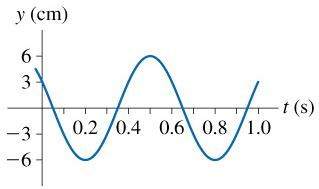# Problem: The figure is a history graph at x=0m of a wave moving to the right at 2m/s.(a) What is the frequency of this wave?(In Hz)(b) What is the wavelength of this wave?

###### FREE Expert Solution

Velocity, frequency, and wavelength relation:

$\overline{){\mathbf{v}}{\mathbf{=}}{\mathbf{f}}{\mathbf{\lambda }}}$

Frequency and periodic time are related as:

$\overline{){\mathbf{f}}{\mathbf{=}}\frac{\mathbf{1}}{\mathbf{T}}}$

1s-1 = 1 Hz

(a)

Periodic time is the time from crest to crest.###### Problem DetailsThe figure is a history graph at x=0m of a wave moving to the right at 2m/s.

(a) What is the frequency of this wave?(In Hz)

(b) What is the wavelength of this wave?